Published: 15 May 2014

# Relation between reduction of weighted impact sound pressure level and shape of small size specimen of floating floor construction

Vidmantas Dikavičius1
Kęstutis Miškinis2
Karolis Banionis3
Edmundas Monstvilas4
1, 2, 3, 4Institute of Architecture and Construction of Kaunas University of Technology, Kaunas, Lithuania
Corresponding Author:
Vidmantas Dikavičius
Views 85

#### Abstract

It is very convenient to evaluate new floating floor constructions by using a small size specimen ($<$1 m2) in real building instead of testing the same construction in a laboratory on a big area ($\ge$10 m2) specimen (as it is required by the standard). No information has been found in scientific literature about any research in which such small specimens (for example, 0.5 m2) were used. Therefore a question occurred whether such specimens could in fact be used in practice instead of big ones ($\ge$10 m2) and if so, under what conditions. In order to answer this question, the first step is to study the previous research. It provides the $∆{L}_{W}$ values of small size specimens and how they differ (are smaller) from those of big specimens. As a result of this, a new question has arisen whether the shape (the ratio of the sides of a rectangular specimen) of the specimen does have an influence on the results ($∆{L}_{W}$) or whether only the size of the specimen (area) influences it. In this experiment, the specimen shape was changed from rectangular to square (though maintaining the same specimen’s area of about 0.5 m2). The results of the experiment showed that different shapes of a small specimen have negligible influence on $∆{L}_{W}$ value. Moreover, the experiment results, which provided some answers to previously mentioned questions, led us to offer evaluation methodology for determination and compensation of $∆{L}_{W}$ measurement methodology errors, which are hypothetically independent from physical parameters of floating floor construction components using small area (0.5 m2) specimens. In order to be sure of the correctness of the presented methodology, a reinforcement of further investigation is required.

## 1. Introduction

For assurance of required impact sound insulation (as showed in practice [1-5]), the best way is to use floating floor constructions. The acoustical performance of such a floor strongly depends on the construction features of upper layers installed on the base floor (usually a concrete slab). The term of weighted reduction of impact sound pressure level $\mathrm{\Delta }{L}_{W}$ (dB) is used to evaluate an improvement that the floating floor construction has on floor impact sound insulation. $\mathrm{\Delta }{L}_{W}$ (dB) value shows how much impact sound pressure level reduces by adding an upper layer to the bare base concrete floor (with respect to that of the bare base floor). To determine this improvement ($\mathrm{\Delta }{L}_{W}$), it is necessary to perform measurements in the laboratory according to the requirements of LST EN ISO 10140 series standards [6-9]. It is required to use a large-scale specimen with an area of $\ge \text{10 m}\text{2}$. However, to use large specimens is very inconvenient due to expensive production and the terms of production. It would be much more preferable [10-16] to use a small size specimen (area 0.5 m2) instead of a large ($\ge \text{10 m}\text{2}$ area) one because of these reasons. It is also important that such measurements could be performed in real buildings where the conditions are similar to those in the laboratory. The advantage of using small specimens is the quick evaluation of different floating floor constructions. On the other hand, as the experiment showed , the use of small specimens provides methodology errors. As it was determined, a small size specimen gives significant errors of the determined value of reduction of impact sound pressure level $\mathrm{\Delta }{L}_{w}$ with respect to that of a big area specimen (10 m2). Another question arises whether only the area of the specimen is responsible for the measurement error and to what extent the square-like specimen form (side ratio) could influence that error (while the area of the specimen remains nearly the same). A comparison of determined $\mathrm{\Delta }{L}_{w}$ values of different shape specimens was done. The results showed that specimens’ shapes do not have significant influence on the determined value. For this research, a heavyweight floating floor construction has been chosen. Such floor construction consists of a sand/cement screed and a resilient material layer of mineral wool. It gave an idea to explore whether $\mathrm{\Delta }{L}_{w}$ determination error depends (if so, to what extent) on the shape of a small specimen.

## 2. Methods and equipment

The measurements are done according to LST EN ISO 10140 series standards. The experiment is performed in the following sequence:

1) Measurement of normalized impact sound pressure level ${L}_{n}$ of bare base floor;

2) Measurements of normalized impact sound pressure levels of different shape small area specimens of floating floor laid on base floor;

3) Determination of weighted reduction of impact sound pressure level $\mathrm{\Delta }{L}_{w}$ of used specimens;

4) Comparison of measurements’ results.

Wireless building acoustics system Nor1516 (“Norsonic”) is used for the measurements. The impact sound pressure levels (${L}_{n}^{\text{'}}$) are measured in one-third octave bands in the frequency range from 100 to 5000 Hz. Standard tapping machine Nor211A (“Norsonic”) is used as the impact sound source. All the measurements are performed and the acquired data is evaluated according to the requirements of LST EN ISO 140-7:2001 and the standards of LST EN ISO 717-2:2001. The standard deviation of the measured impact sound pressure level is up to 4 dB in 100-160 Hz frequency range and up to 2 dB in 200-5000 Hz frequency range.

The research is performed in a real building. Two rooms (located one above the other) are chosen for the test (Fig. 1).

Fig. 1Test rooms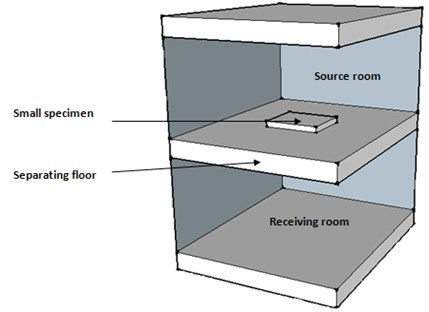The source room has a bare base floor without any coverings. All constructions of the chosen building are heavyweight concrete columns and walls ($\ge$350 kg/m2 mass per unit area). It was considered that flanking sound transmission there was insignificant. Firstly, the impact sound pressure level of the base concrete floor is measured in the middle of the room inside of the marked rectangular of the dimensions of 1000×1000 mm (Fig. 2). Only one position is chosen because this measurement is comparative.

Afterwards, five different specimens (described below) put on the same spot are measured. The measurements are done the same day so to assure the same testing conditions. The testing is performed in the same room as the previous experiment  evaluating the influence that specimen’s size has on weighted reduction of impact sound pressure level $\mathrm{\Delta }{L}_{w}$.

Fig. 2Plan of the source room: 1 – marked rectangular; 2 – test specimen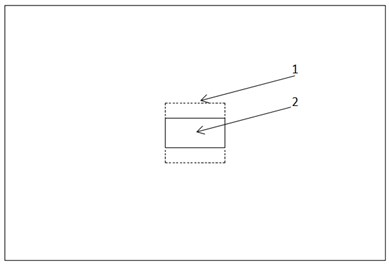The small size element of the floor (0.5 m2) is tested. In order to evaluate shape influence on weighted reduction of impact sound pressure level $\mathrm{\Delta }{L}_{w}$, five different specimens (sand/cement screed elements) with following dimensions are prepared and tested: “A” (1000×500 mm); “B” (900×550 mm); “C” (800×600 mm); “D” (750×650 mm) and “E” (700×700 mm) (showed in Fig. 3). The ratios of the specimens’ sides are respectively: A – 2; B – 1.8; C – 1.3; D – 1.2; E – 1.

Fig. 3Specimens used for the test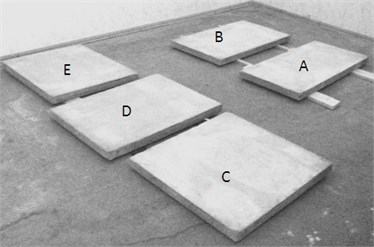Fig. 4The cross-section of the specimen on the base floor: 1 – 50 mm thick send/cement screed element; 2 – 50 mm thick mineral wool; 3 – 220 mm thick hollow concrete slab.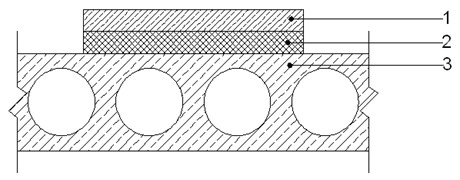The mass per unit area of the slab of the base concrete floor is 350 kg/m2. Commonly used stone wool sheet (50 mm thick and 119 kg/m3 dense) is laid on the base floor under the specimen. The same sheet is used under all the specimens. The dynamic stiffness of the stone wool is measured in the laboratory according to LST EN 29052-1 standard and is equal to 18 MN/m3. The specimens – elements of commonly used sand/cement screed – are produced in the laboratory and hardened for 28 days at 20°C temperature in 100 % humidity (in water). Afterwards, the specimens are moved and kept in the testing (source) room for 7 days before performing measurements. All five specimens are 50 mm thick and have about 110 kg/m2 mass per unit areas (Fig. 4). The compressive strength of sand/cement screed is about 30 MPa. The mass per unit area and compressive strength of the specimens are determined from hardened cubes made from the same mixture.

Only one tapping machine position (in the center of the specimen) is used due to small size of the specimen (Fig. 5).

Fig. 5The view of the source room with a small rectangular specimen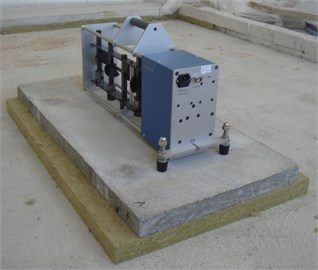The edges of the specimens are left un-damped (Fig. 5). The measurements are performed using the same specimens with only one difference: their edges are covered with special hard weight mastic (Perannator) in order to check whether damping has any influence on the results (Fig. 6).

Fig. 6a) Photo of a small specimen with dampened edges; b) cross-section of a damped specimen c) plan view of a damped specimen. 1 – damping material (Perannator); 2 – sand/cement screed (test element); 3 – mineral wool; 4 – hollow concrete slab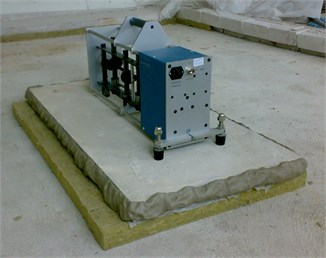a)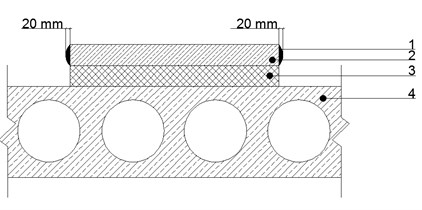b)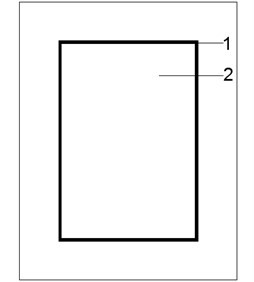c)

Five microphone positions are used for the measurement of impact sound pressure level in the receiving room. The duration of each measurement is 6 seconds. The average value is calculated from these five measurements.

## 3. Results and discussion

Measurements and evaluation of the results are done as described in previous chapters. The comparison of normalized impact sound pressure levels (average values) of different shape specimens (“A”-“E” type) is given in Fig. 7. The results (Fig. 7) demonstrate that the character of impact sound pressure level curves is similar but places of resonances (level peaks) in low and mid frequency ranges depend on the specimen’s shape (the ratio of different sides). For “A” type specimen the resonances occur at 200 Hz and 630 Hz, for “B” – 250 Hz and 630 Hz, for “C” – 315 Hz and 630 Hz, “D” – 500 Hz and “E” – 400 Hz. This shows that when changing specimen form from rectangular to square the resonances move from low frequencies to mid range. In high frequency range (from 2000 Hz) the curves are smooth of all specimens. This occurs due to the changed bending wave reflection conditions from the edges of the specimens.

Normalized weighted impact sound pressure level ${L}_{n,w}^{\text{'}}$ is calculated using data of the measurements presented in Fig. 7 – a single parameter of impact sound insulation. The comparison of ${L}_{n,w}^{\text{'}}$ values (from different shape specimens) are presented in Fig. 9. The difference of values is up to 3 dB for different types of specimens. This means that the form of the specimen does not have significant influence on the impact sound pressure level. Therefore a conclusion can be made: when a small specimen is used to test floating floor constructions, the ratio of the specimen’s edges is not important.

Fig. 7Comparison of normalized impact sound pressure levels of different shape specimens (uncovered edges)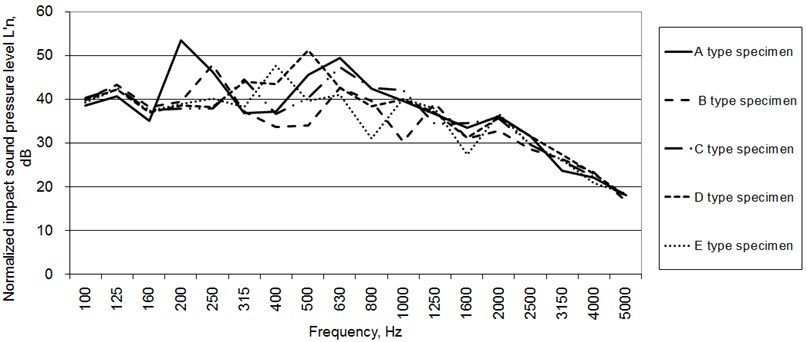The comparison of impact sound pressure levels of the same specimens with covered (damped) edges is given in Fig. 8. Fig. 8 demonstrates that the character of the impact sound pressure level curves is similar to those of the specimens with uncovered edges. The difference is that the amplitude of resonances is less expressed but appears at the same frequencies. Moreover, the impact sound pressure level decreased at the average of about 5 dB. This means that damping helps to reduce resonances and impact sound pressure levels due to the mass added to specimen’s edges. This decrement shows that when using small specimens it is better to damp their edges with high-density amorphous material (for example, Perranator). This presumably enables to reduce boundary influence on the results. By stating that, we keep in mind that damping specimen’s edges helps to approach banding wave-propagating conditions (close to those existing in a big specimen), and the impact sound pressure level will depend purely on the area of the specimen.

Fig. 8Comparison of normalized impact sound pressure levels of specimens of different shapes (edges covered with mastic)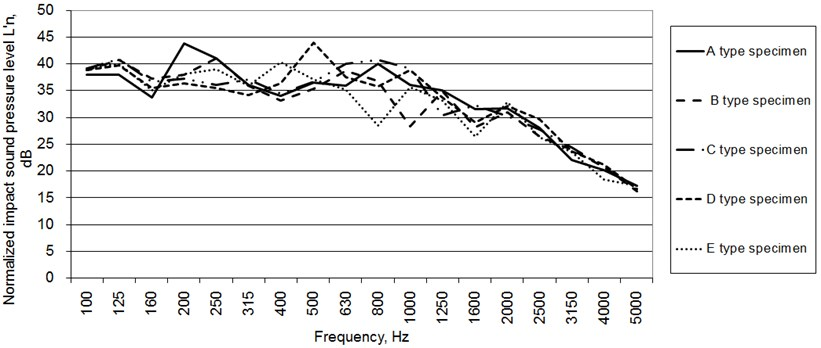Normalized weighted impact sound pressure level ${L}_{n,w}^{\text{'}}$ values are calculated using the curves of ${L}_{n}^{\text{'}}$, (given in Fig. 7 and Fig. 8) for specimens with uncovered and covered edges respectively. The comparison of these values of different shape specimens is given in Fig. 9 and Fig. 10 respectively. This comparison is presented in order to show how the changes of the shape influence the value of a single parameter ${L}_{n,w}^{\text{'}}$. It is difficult to draw any conclusions from the measurement curves due to different shapes of the curves. This comparison demonstrates that the shape of the specimen does not significantly influence the value of weighted normalized impact sound pressure level.

Fig. 9The comparison of Ln,W' values of different shape specimens with uncovered edges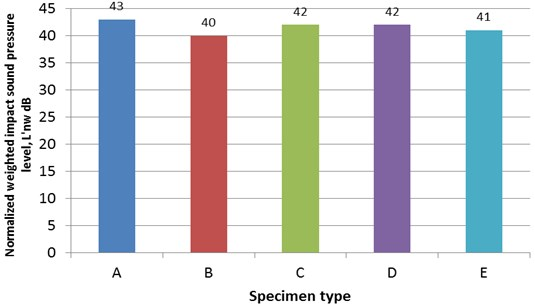The dependence of methodology error of $\delta \mathrm{\Delta }{L}_{W}$ of determination of $\mathrm{\Delta }{L}_{W}$ value from the area of the specimen being tested can be approximated by the following expression (by using previous data, the data of this experiment and referring to the logarithmical dependence of radiated vibration power from the radiating surface area ):

1
$\delta \mathrm{\Delta }{L}_{W,app}=10\mathrm{l}\mathrm{g}\left(\frac{{S}_{0}}{S}\right),$

where $S$ is the screed area of the small size specimen being measured in m2, ${S}_{0}$ is the reference area equal 10 m2 with respect of which the value of $\delta \mathrm{\Delta }{L}_{w,app}$ is normalized.

Therefore the true value of the weighted reduction of impact sound pressure level $\mathrm{\Delta }{L}_{w,cor}$ (equal to $\mathrm{\Delta }{L}_{w}$ value corresponding to the area of the 10 m2 specimen) of the floor with a floating sand-cement screed (using a small size specimen) is calculated by the following formula:

2
$\mathrm{\Delta }{L}_{W,cor}=\mathrm{\Delta }{L}_{W}=\mathrm{\Delta }{L}_{W,s}-\delta \mathrm{\Delta }{L}_{w,app}=\mathrm{\Delta }{L}_{W,s}-10\mathrm{l}\mathrm{g}\left(\frac{{S}_{0}}{S}\right),$

where $\mathrm{\Delta }{L}_{W,s}$ is the weighted reduction of impact sound pressure level, which is determined by using a small size specimen with an area less than 10 m2.

Fig. 10The comparison of Ln,W' values of different shape specimens when edges are covered with mastic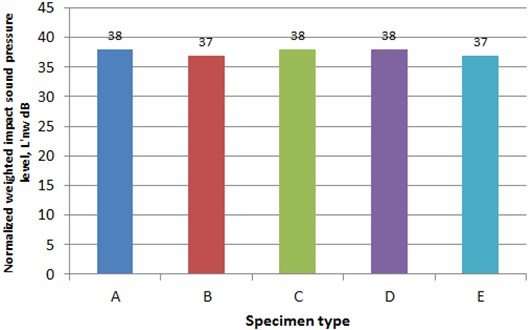Fig. 11Comparison of impact sound pressure levels of “B” type specimens when edges are un-damped and damped with mastic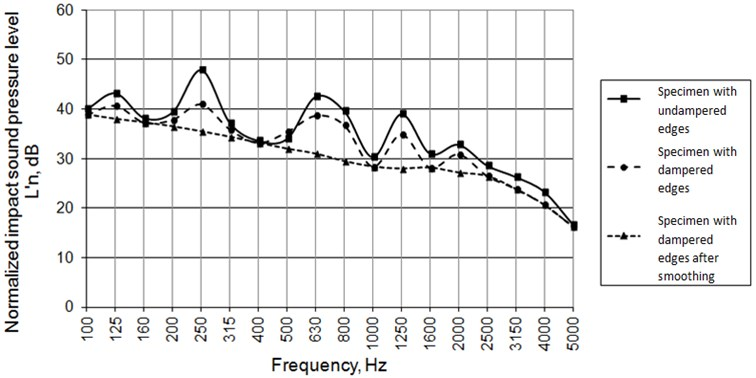In order to check the actuality of this assumption, we used data from article . Repeatedly, the values of experimentally determined methodology errors  and the ones approximated by a Eq. (1) are presented in Fig. 12.

A noticeable difference of 2 dB exists between the values determined from measurement data (point “2”) and the one calculated according to Eq. (2) (point “1”) only for the specimen with area $S=$0.5 m2. It can be assumed that this mismatch is conditioned by the resonance peaks in ${L}_{n,W}^{\text{'}}$ spectra curve.

As it is known from the previous experiment , a small size specimen provides a methodology error $\delta \mathrm{\Delta }{L}_{W}$ of determination of weighted reduction of impact sound pressure level $\mathrm{\Delta }{L}_{W}$ with respect to that specimen of an area of 10 m2. The analysis of the graph has yield an impression that in order to acquire the value to be closer to the true value the resonances should be eliminated. For this purpose, the so-called “smoothing operation” can be used. The example of such operation is given in Fig. 11. The “B” type specimen’s measured values (curves) are chosen for this purpose. After “eliminating” resonances, the single value of impact sound pressure level ${L}_{n,W}^{\text{'}}$ is equal 34 dB and the correspondent to it $\mathrm{\Delta }{L}_{W}$ value is equal 38 dB (determined from the data of measurement).

Fig. 12“Error” values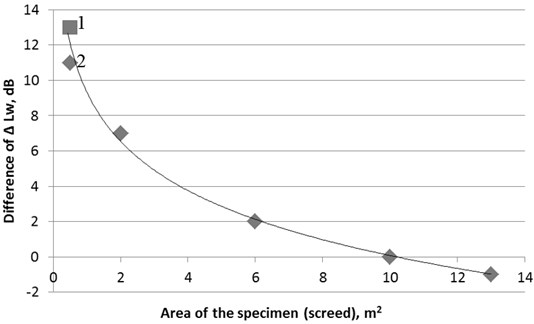After the resonance peaks are artificially and graphically “smoothed”, a full coincidence of values yield from the measurement data and calculated data. With regards to this coincidence, a hypothesis is made that for any type of a rigid floating floor system, which contains rigid screeds on any resilient interlayer, the error $\delta \mathrm{\Delta }{L}_{w}$ (using small area rigid floating screed specimens on heavyweight a concrete base floor always) will be the same and equal to that $\delta \mathrm{\Delta }{L}_{W,app}$ calculated by Eq. (2) e. g. invariant to the absolute values of ${L}_{n}^{\text{'}}$ and the respective value of $\mathrm{\Delta }{L}_{w}$, and depends only on the area of the screed. Additional tests on different rigid floor screeds and resilient underlies have to be done in order to warrant the hypothesis.

## 4. Conclusions

1. The results of the research reveal that shape of the test specimen (sand/cement screed) has insignificant influence on the test results. The difference is up to 3 dB of normalized weighted impact sound pressure level ${L}_{n,W}^{\text{'}}$. The frequencies of the resonances depend on the shape of the specimen.

2. The damping of the specimens by adding extra mass to their edges reduces normalized impact sound pressure level ${L}_{n}^{\text{'}}$. Moreover, it reduces the value of weighted normalized impact sound pressure level ${L}_{n,W}^{\text{'}}$ with respect to the not damped ones in average of 4 dB.

3. The presented logarithmic formula could be used for the determination of $\delta \mathrm{\Delta }{L}_{W,app}$ when measuring on a small size specimen ($<$10 m2) in a real building.

4. The use of “smoothing operation” enables to eliminate resonances and to get “the real value” of $\mathrm{\Delta }{L}_{W}$ when a small size specimen ($<$10 m2) of the analyzed floor construction is used.